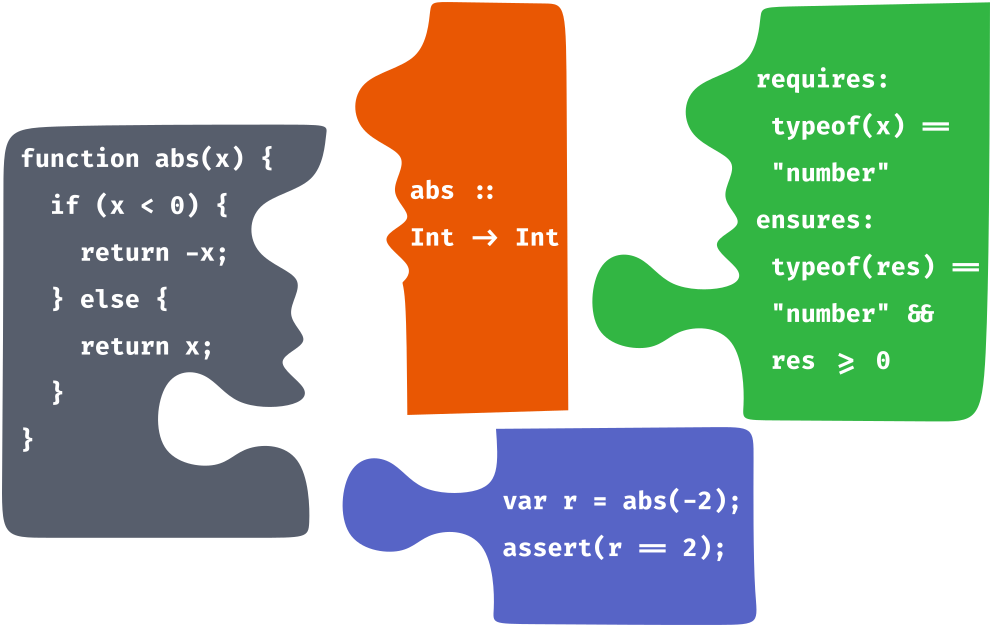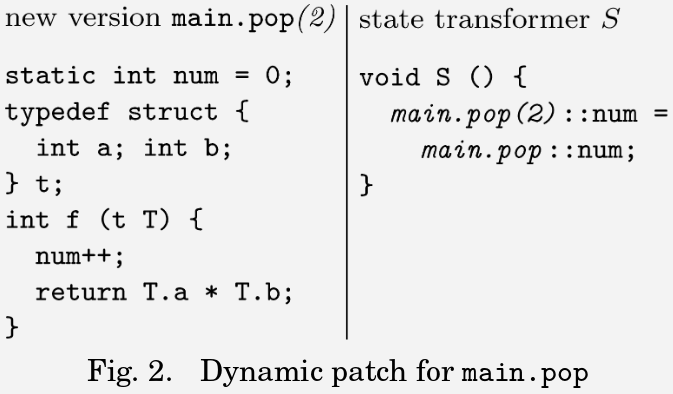# Towards Live Programming Environments for Statically Verified JavaScript esverify.org/thesis Christopher Schuster University of California, Santa Cruz cschuste@ucsc.edu

## Motivation

Technology should not aim to replace humans, rather amplify human capabilities.
– Douglas Engelbart, 1958

## Introduction

• A computer is a universal machine that can process any kind of information and perform any kind of computation
• Computation and steps are specified with a programming language
• very precise and technical
• unlike natural languages
• Programming is often tedious, frustrating and error-prone!

## Program edits and feedback loopProgramming is an iterative cycle of code edits and feedback until the program behavior matches the intended behavior

Live programming environments improve this feedback cycle by enabling programmers to edit the code of running programs and obtain immediate feedback

## Tests, Types and Program VerificationCheck whether multiple specifications are consistent with each other and provide feedback about discrepancies

## Outline

• Live programming
• Live feedback for graphical applications based on a certain programming style
• Live programming by example/direct manipulation
• Program Verification
• Static verification of dynamically-typed JavaScript, including higher-order functions
• Integrated Development and Verification Environments

## Live Coding

An art or music performance in front of an audience (see e.g. Sam Aaron and Ben Smith)

## Dynamic Software UpdatingPatching running systems with explicit state migrations (see e.g. Michael Hicks and Scott Nettles)

## Self-contained Development EnvironmentsEnvironments with integrated development tools and runtime code updates (see e.g. Squeak Smalltalk)

## Live Programming

Runtime code updates during the development process with explicit emphasis on immediate feedback (see e.g. Elm Debugger)

## Live Programming

If there is any delay in the feedback loop between thinking of something and seeing it, then there is this whole world of ideas which will never be.
– Bret Victor

## Live Programming for GUI Applications

• Single-threaded event loop (e.g. JavaScript)
• No code updates during events
• Pure Rendering Function to safely refresh output after each code update
• Closures do not persist between events

 1
2
3
4
5
6
7
8
9
<span id="val">Count: 0</span>
<button id="incButton">+</button>
<script>
var count = 0;
$("#incButton").on("click", function () { count++;$("#val").html("Count: " + count);
});
</script>
Count: 0

Problem: No live feedback for changes due to the structure of the code

## Model-View-Update Pattern (MVU)


let count = 0;

function view() {
return (
<div>
Count: {count}
<button onclick={inc}>
Inc!
</button>
</div>);
}

function inc() {
count++;
}
• Model holds entire application state
• View generates visible output based on Model
• Update processes events and changes the Model

## Dynamic String-Origin Tracking

String literals in the source code are wrapped in transparent proxies that track string origin information

let x = "ab";       x: [ ("ab", 9) ]
let y = x + "c";    y: [ ("ab", 9), ("c", 16) ]
let z = y;       z: [ ("b", 10) ]

More complex programming-by-example introduces ambiguities

• Need heuristics/ranking or user interactions!

## Summary

Event-based MVU architecture programming model enables
• live programming of the UI code,
• back-in-time debugging, runtime version control and
• live programming by example.

## Outline

• Live programming
• Live feedback for graphical applications based on a certain programming style
• Live programming by example/direct manipulation
• Program Verification
• Static verification of dynamically-typed JavaScript, including higher-order functions
• Integrated Development and Verification Environments

## Tests, Types and Program VerificationCheck whether multiple specifications are consistent with each other and provide feedback about discrepancies

## Automatic Unit Testing

• Testing only covers a certain set of example inputs

## Type Checking

• Types are often not precise enough
• Dynamically-typed programming idioms not well supported

## Program Verification

• Verification of pre-, postconditions and invariants often requires annotations and hints to the verifier

## JavaScript Verification with esverify

• Pre- and postconditions, etc. written as standard boolean JavaScript expressions
• Annotations are used for static verification and removed before execution
• Instead of type syntax, the typeof operator can be used
• Need to manually supply loop invariants and other verification hints

## Another Verification Example

• Conjunction, disjunction and negation in logical annotations similar to union and intersection types

## Verification Conditions

• Assertions are translated to logic and checked by SMT solving

## Verification Conditions


function max(a, b) {
requires(typeof(a)=="number");
requires(typeof(b)=="number");
ensures(result => result >= a);

if (a > b) {
return a;
} else {
return b;
}
}

$\forall a \forall b.$
$~~~~~~\,\texttt{typeof}(a) = number''$ $\wedge~~~~\texttt{typeof}(b) = number"$

$\wedge~~~~a > b~\Rightarrow~result\negmedspace=\negmedspace a$

$\wedge~~~~\lnot (a > b)~\Rightarrow~result\negmedspace=\negmedspace b$

$\Longrightarrow result \ge a$

## Challenges for Verification:Loops and Recursion

• Loops and recursion lead to unbound number of paths
• Inferring loop invariants and post-conditions undecidable
• Explicit postconditions and invariants required

## Loops: Sum of Natural Numbers


function sumTo (n) {
requires(Number.isInteger(n));
requires(n >= 0);
ensures(res => res === (n + 1) * n / 2);

let i = 0;
let s = 0;
while (i < n) {
invariant(Number.isInteger(i));
invariant(Number.isInteger(s));
invariant(i <= n);
invariant(s === (i + 1) * i / 2);
i++;
s = s + i;
}
return s;
}


## Function Definitions and Calls


function max(a, b) {
requires(typeof(a)=="number");
requires(typeof(b)=="number");
ensures(result => result >= a);

if (a > b) {
return a;
} else {
return b;
}
}

const x = max(23, 42);
assert(x >= 23);


$\forall x.$

$~~~~ ( \forall a, b.~($

$~~~~~~ \texttt{typeof}(a) = \texttt{"number"} ~\wedge~$

$~~~~~~ \texttt{typeof}(b) = \texttt{"number"} ~\Rightarrow~$

$~~~~~~ max(a, b) \ge a ) )$

$\wedge ~~~~ x = max(23, 42)$

$\Longrightarrow x \ge 23$

## Function Definitions and Calls: Details

• The pre- and postcondition of a function can be referred to with the uninterpreted construct $\texttt{pre} (f, x)$ and $\texttt{post} (f, x)$
• A custom bounded trigger-based quantifier instantiation algorithm is used:
• Function calls act as triggers for a limited number of instantiations
• Avoids brittle instantiation heuristics, matching loops and SMT solver timeouts

## Quantifier Instantiation (1/3)


function inc (x) {
requires(Number.isInteger(x));
ensures(y => Number.isInteger(y) && y > x);
return x + 1;
}


$\forall x. \{ call(x) \} \Rightarrow$

$\left( isInt(x) ~\Rightarrow~pre(\texttt{inc}, x) \right) ~\wedge~ \left( isInt(x) \wedge \,post(\texttt{inc}, x)~\Rightarrow~ \texttt{inc}(x) > x \right))$

• $\{ call(x) \}$ is a trigger pattern
• $pre(\texttt{inc}, x)$ and $post(\texttt{inc}, x)$ uninterpreted function symbols
• $pre(\texttt{inc}, x)$ is satisfied by any integer value for $x$
• $post(\texttt{inc}, x)$ and precondition together imply $\texttt{inc}(x) > x$

## Quantifier Instantiation (2/3)


function inc (x) {
requires(Number.isInteger(x));
ensures(y => Number.isInteger(y) && y > x);
return x + 1;
}
const y = inc(23);
assert(y > 23);


$\forall y.$

$~~~~~~ \left( \forall x. \{ call(x) \} \Rightarrow \left( ...~\Rightarrow~pre(\texttt{inc}, x) \right) \wedge~ \left( ... \wedge \, post(\texttt{inc}, x) ~\Rightarrow~ ... \right) \right)$

$\wedge ~~~~ call(23)$

$\wedge ~~~~ post(\texttt{inc}, 23)$

$\wedge ~~~~ y = \texttt{inc}(23)$

$\Longrightarrow y > 23$

## Quantifier Instantiation (3/3)


function inc (x) {
requires(Number.isInteger(x));
ensures(y => Number.isInteger(y) && y > x);
return x + 1;
}
const y = inc(23);
assert(y > 23);


$\forall y.$

$~~~~~~\, \left( isInt(23) ~\Rightarrow~pre(\texttt{inc}, 23) \right)$

$\wedge~~~~ \left( isInt(23) ~\wedge~post(\texttt{inc},23)~\Rightarrow~ \texttt{inc}(23) > 23 \right)$

$\wedge ~~~~ post(\texttt{inc}, 23)$

$\wedge ~~~~ y = \texttt{inc}(23)$

$\Longrightarrow y > 23$

## Higher-order Functions: Twice


function inc (x) {
requires(Number.isInteger(x));
ensures(y => Number.isInteger(y) && y > x);
return x + 1;
}

function twice (f, n) {
requires(spec(f, (x)   => Number.isInteger(x),
(x,y) => Number.isInteger(y) && y > x));
requires(Number.isInteger(n));
ensures(res => res >= n + 2);
return f(f(n));
}

const m = twice(inc, 3);
assert(m >= 5);


## Challenges for Verification:Recursive Data Types

• Nested recursive data structures (records, lists, trees)
• Proposed Solution: Simple immutable "classes" with invariants
• Check invariant when creating instances
• Instantiate invariant when accessing properties


constructor (base) {
this.base = base;
}
invariant () {
return Number.isInteger(this.base);
}
requires(Number.isInteger(n));
return this.base + n;
}
}

$\forall x. \{ access(x) \} \Rightarrow \left( ~x ~~\texttt{instanceof} ~~ \texttt{Adder} ~\Rightarrow~ isInt(x.base) \right)$

## Case Studies

• Reversing an ascending list yields a descending list
• A verified implementation of MergeSort
• A custom list class that can be parameterized by an invariant (somewhat analogous to generics)
• A theorem and proof written in JavaScript showing that any locally increasing integer-ranged function is globally increasing

About 50-80% of source code are verifier annotations

## Formal Development with $\lambda^S$

• Pure functional core language with logical propositions as pre- and postconditions
• Verification rules similar to typing rules but also generate verification conditions
• Verification is sound:
• Verified programs execute without getting stuck
• Verification algorithm is decidable
• Decision procedure terminates for all inputs
• Complete mechanized proof in Lean

## Refinement Typing with $\lambda^T$

$T \in \text{Types} ~::=~ \{~x: B~|~ R~\} ~|~ x:T \rightarrow T \hspace{2.5em} B ~::=~ \text{Bool}~|~ \text{Int}$

• Refinement types translate to annotations
• Dependent function types translate to spec
• Conjuecture that well-typed programs translate to verifiable programs
• Proof is work in progress

## Summary

• Static program verification can be applied to dynamically-typed JavaScript programs including higher-order functions
• A custom quantifier instantiation ensures that the verification process remains predictable
• Verification is sound and (seemingly) at least as expressive as refinement type systems such as LiquidHaskell

## Outline

• Live programming
• Live feedback for graphical applications based on a certain programming style
• Live programming by example/direct manipulation
• Program Verification
• Static verification of dynamically-typed JavaScript, including higher-order functions
• Integrated Development and Verification Environments

## Verification Issues and Tool Support

• Verification issues can be hard to understand and fix due to expressive logic and complex verification procedure
• Explain verification issues with executable counterexamples (tests)
• An integrated Verification Inspector can show details about a verification issue and enables interactive exploration

## Counterexamples and Test Generation

• Can automatically generate test based on original code and counterexample values
• Annotations become dynamically-checked assertions in the test
• For higher-order functions, this includes synthesis of function values and contract checking

## User Study

• Evaluated the development environment with verification integration with an online user study with 18 participants
• User study involved:
• a tutorial,
• a series of programming and verification tasks, and
• a survey.
• Archived user study:
esverify.org/userstudy-archived

## User Study Results

Response (%) Verification Inspector Counterexample Popups Integrated Debugger
UI Issues 50 39 44
Not useful 6 6 6
Impairs development 11 0 6

## Summary

• Verification errors can be difficult to understand
• Executable counterexamples and tool integration can assist programmers
• A novel Verification Inspector enables programmers to interactively add or remove assumptions and assertions
• Features are generally seen as helpful by user study participants

## Conclusions

To improve our collective ability to solve the world’s problems, we must harness the immense promise and power of technology.
– Douglas Engelbart

## Conclusions

• To solve problems with technology, programmers need to communicate the intended program behavior
• Independent of future advances in artificial intelligence
• Programming is an iterative process
• Programming environments should aim to provide immediate live feedback for any change to the code
• Static verification enables programmers to specify detailed invariants and check the implementation
• Interactive tools and environment integration is crucial to make verification usable in practice

# Thank you for your attention! Questions?esverify.org/thesis

## User Study Results (2/2)

Response (%) Verification Inspector Counterexample Popups Integrated Debugger
Used this feature in experiments 39 50 28
Unsuccessfully tried using it 33 33 22
Did not use it 27 17 50

/